# My first extension

This my first extension name Calculator [Free]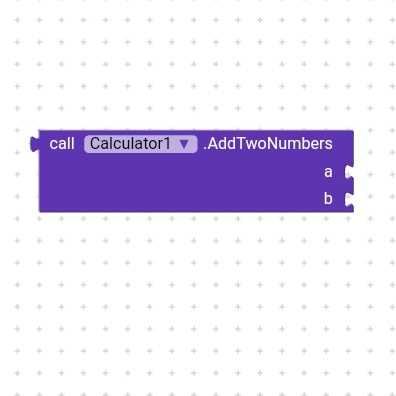You can add two numbers with this block. a = number, b = number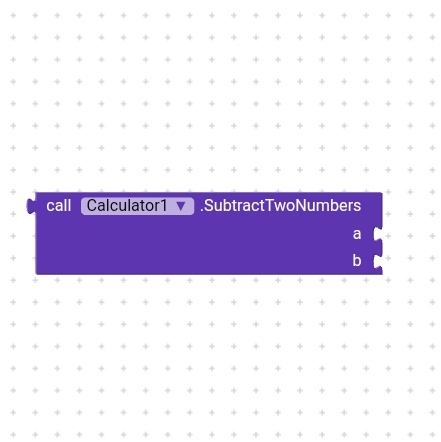You can subtract two numbers with this block. a = number, b = number
Note: a > b else it gives value in -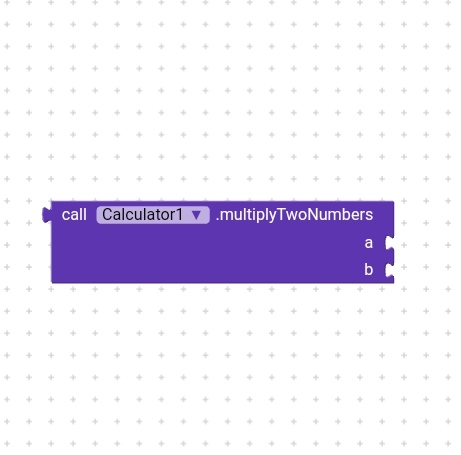You can multiply two numbers with this block. a = number, b = number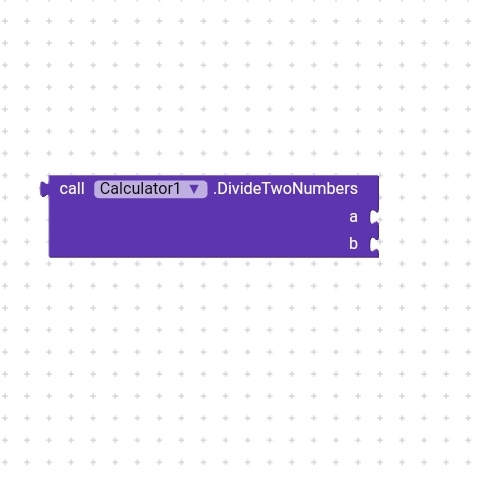You can divide two number with this block. a = number, b = number
Note: a > b else it gives 0

You can find dividend with this block. Divisor = number, Quotient = number, Remainder = number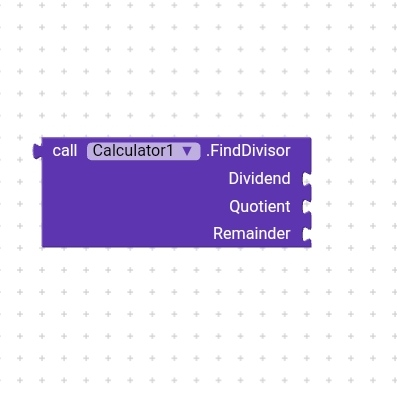You can find divisor with this block. Dividend = number, Quotient = number, Remainder = numberYou can find remainder with this block. Dividend = number, Divisor = number, Quotient = number

3 Likes

Good startFrom now you will have to work hard to create unique and useful extensions.
All the best for that.

However, make sure to follow

2 Likes

Ok thanks for help I work hard

1 Like
2 Likes